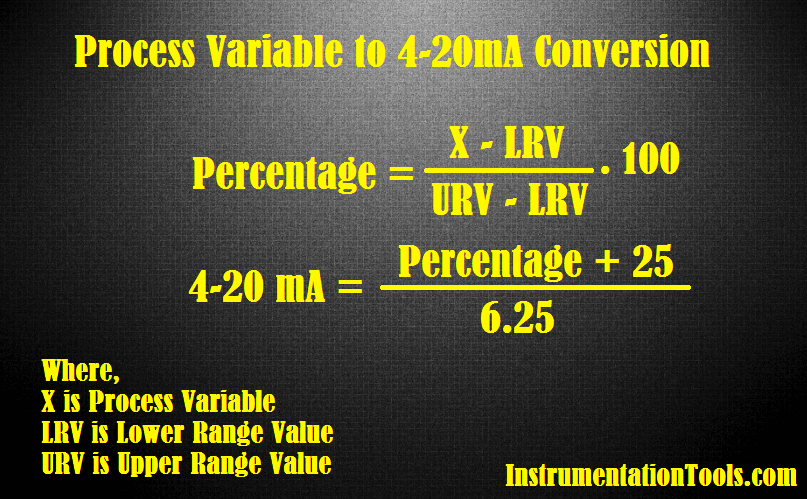# Formula to Calculate 4-20 mA from Process Variable

How to Calculate 4-20mA current loop from Process Variable value of a field transmitter. Check the formula to find out equivalent 4-20mA signal from Process Variable.

## Calculate 4-20mA from Process Variable##### Formula :

First calculate equivalent percentage from the process variable

#### Percentage = ( X – LRV / URV – LRV ) . 100

Then calculate equivalent 4-20mA signal from percentage

#### 4-20mA = ( Percentage + 25 ) / 6.25

Where,

X is Process Variable

LRV is Lower Range value

URV is Upper Range Value

Example:

#### A Flow transmitter has a range of 0 to 2500 m3/hr and it is displaying flow rate of 1500 m3/hr. calculate the transmitter output current.

Process variable = 1500 m3/hr

Lower Range Value, LRV = 0 m3/hr

Upper Range Value, URV = 2500 m3/hr

Note: units must be same for process variable, LRV & URV

Percentage = (1 500 – 0 / 2500 – 0 ) . 100 = 60 %

4-20 mA Signal  = (Percentage + 25) / 6.25 = (60 + 25) / 6.25 = 13.6 mA

Also you can use online Instrumentation Calculator for Automatic calculations. Click Here.

#### Articles You May Like :

0 to 100% to Process Variable

4-20 mA to 0 to 100% Formula

Flow Transmitter Output Value

4-20mA Fraction of Measurement

Remote Seals Level Calculation

Don't Miss Our Updates
Be the first to get exclusive content straight to your email.
We promise not to spam you. You can unsubscribe at any time.

### 18 thoughts on “Formula to Calculate 4-20 mA from Process Variable”

1.Thanks for sharing

2.usefull

3.for calculating mA, what is 6.25 value please explain

•Hello Mazhar, 100/(20-4) = 6.25

4.Dear can You explain correctly how 6.25 came. While we are dividing 100/(20-4) it’s not showing 6.25

•Hi, 100/(20-4) = 100/16 = 6.25

5.Very good formula, but how did u derive or get the +25

•Hi, Percentage range is 0 to 100 i.e. starting from zero and current range is 4 to 20 i.e. starting from 4. Thats why we added 25 there. In order to get clear picture, try to calculate equivalent mA value for 0% with & without 25 in the formula.

6.Thanks for the clarification ?

7.Another simple formula for converting Percentage into equivalent 4-20mA current,
4-20mA = (percentage*16)+ 4
Example : 60%*16+4=13.6

8.•Hi, Please see brackets, (60+25)/6.25 = 85/6.25 = 13.6

9.% to MA

%= 60
how much MA at 60%
so
60/6.25+4= 13.6

10.have a one dought about a soot blowing control valve calibartion. Today I done calibartion but it is not full closed valve passing when a giving 0 commond.it is passing 5kg pressure what can I do? Please tell me a prosedure.

11.1500*16=24000
24000/2500=9.6
9.6+4=13.6

12.What is the problem in this calculation…. For 20mA reading is 2500, then for x mA reading is 1500, We calculate x=20*1500/2500= 12mA… What am I missing here

13.direct formula
1500/2500*16+4=13.6ma

14.The LRV (lower range value) will usually be 0 for flows and pressures but not for load cells, drum levels, temperatures, etc.

For example, a -80C freezer may have a temperature transmitter calibrated from -100C to 20C. Formula 1 becomes useful. If the freezer temperature is -70C then what is the ma output from the transmitter?

First, find the transmitter span.

Span is URV – LRV or 20 – (-100) = 120C. Remember SPAN is always ONE number and RANGE is always TWO numbers.

Use the formula at the top to find the percentage:

(-70 – (-100)) / 120 = 30 / 120 = .25 or 25%.

To find the current use one of the formulas above or : (.25 x 16) + 4 = 8 ma